# Python Program to Reverse a linked list

## Introduction

The linked list data structure is a crucial topic to prepare for technical rounds. You can see a variety of ticklish and straightforward questions on this topic. This article will introduce you to the basic problem of a linked list.

To reverse a linked list, you should be clear in your head what a linked list is and how it is implemented in the Python language.

A linked list is a linear data structure where every element acts as a node with two parts data part(which stores the actual data of the element) and the address part(which stores the address of the location of the next node). Linked lists are dynamic, which eases the insertion and deletion of the elements.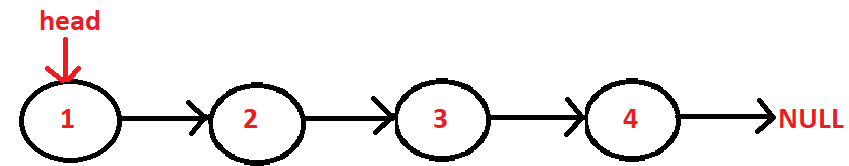Code

``````class Node:
# constructor for defining the data and next
def __init__(self, data = None, next=None):
self.data = data
self.next = next

def __init__(self):

# insertion method
def insert(self, data):
newNode = Node(data)
while(current.next):
current = current.next
current.next = newNode
else:

# print function
def printLL(self):
while(current):
print(current.data)
current = current.next

# Singly Linked List with insertion and print methods
LL.insert(3)
LL.insert(4)
LL.insert(5)
LL.printLL()``````

We are given a singly linked list, and our task is to reverse the linked list by changing the links between the nodes.

Input:Output: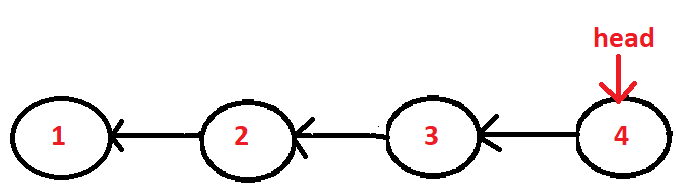Explanation

Here as you can see the list has been reversed.

Now 4->3, 3->2, 2->1.

Now 4 has become the head of the list as it is the starting node of this linked list after performing the reversal action.

Here the only change we can observe is the change in the links. So we have to think of an approach that can change the links between the nodes.

Let’s move to our different approaches to solve this problem.

## Approach1: Iterative

Algorithm

• Create two nodes-> current and next.
• Initially, current(node) will point to the first element(head), and prev will point to null.
• Store the next of current element in next.
• Traverse through the list till the current become null.
• In the end, the prev will be our head.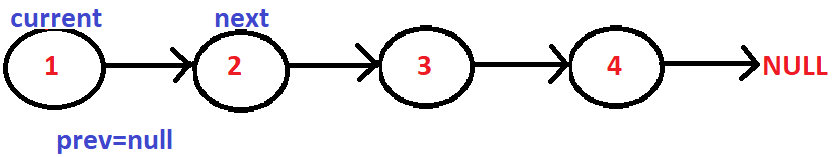The current element will now point to that element stored in the 'prev' variable.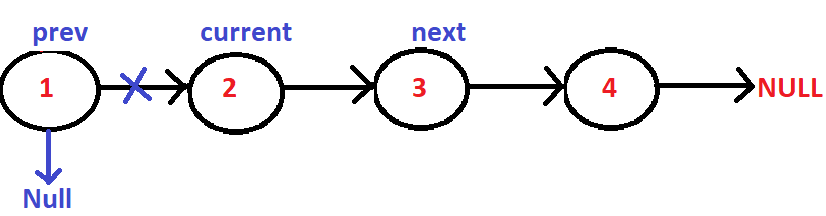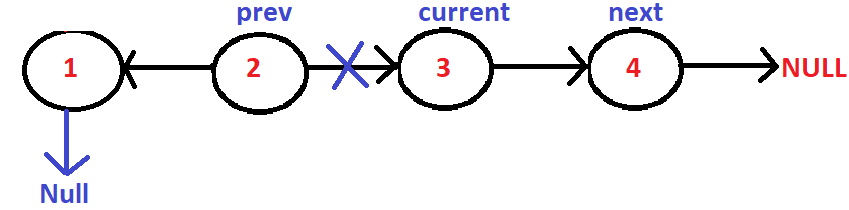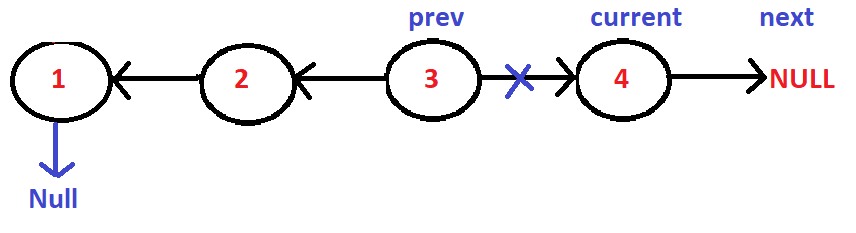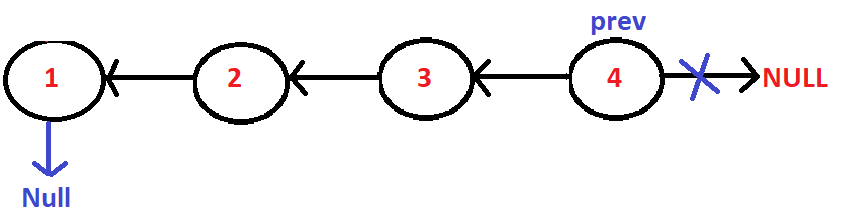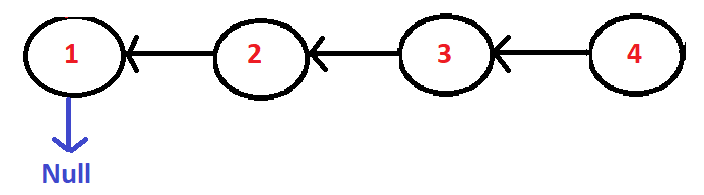## Code

``````class Node:

# Constructor
def __init__(self, data):
self.data = data
self.next = None

def __init__(self):

# To reverse the list
def reverse(self):
prev = None
while(current is not None):
next = current.next
current.next = prev
prev = current
current = next

def push(self, new_data):
new_node = Node(new_data)

# To print the list
def printL(self):
while(temp):
print temp.data,
temp = temp.next

# Main function
llist.push(1)
llist.push(2)
llist.push(3)
llist.push(4)

llist.printL()
llist.reverse()
llist.printL()``````

Output

Time Complexity - O(n).

Space Complexity - O(1).

## Approach2: Recursive

Algorithm

• when the curr node is the last node starts with it and also runs after the previous curr.
•  Save the following curr to next.
•  Next, create from the current point to the previous point.
•  Repeat with curr as curr and curr as prev.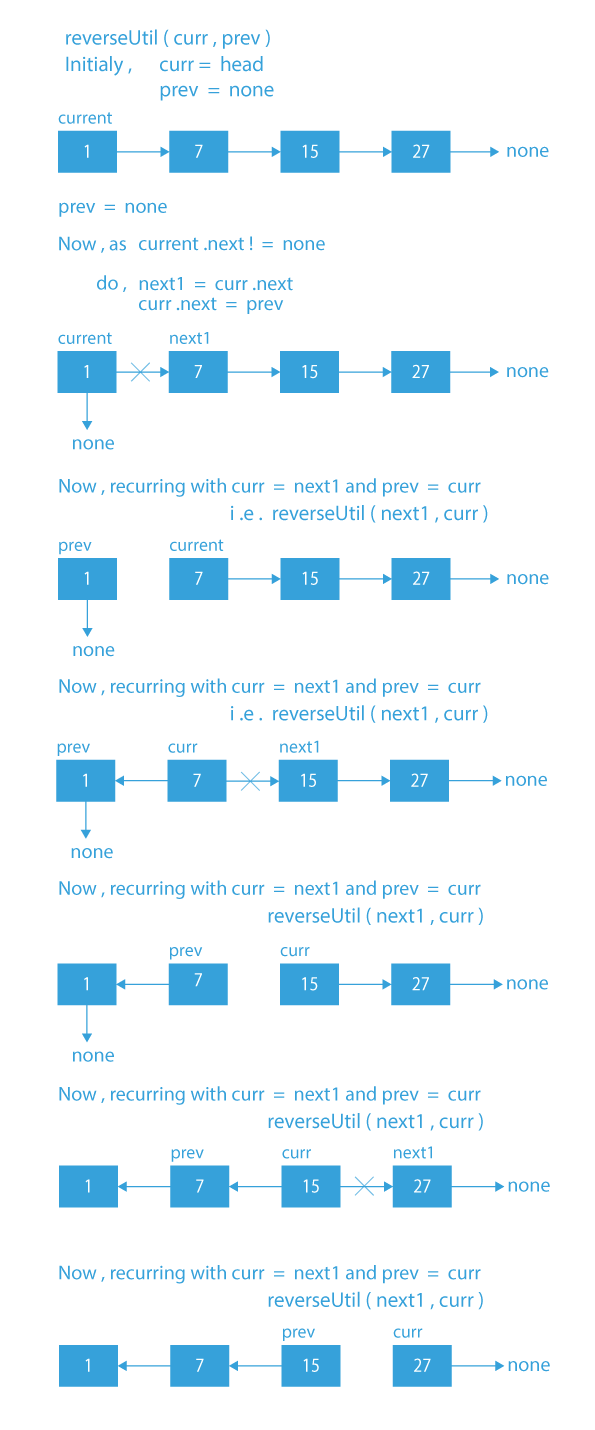Pic referenced from prepbytes.

## Code

``````class Node:

# Constructor
def __init__(self, data):
self.data = data
self.next = None

def __init__(self):

def reverse_Util(self, curr, prev):

# If last node mark it head
if curr.next is None:

curr.next = prev
return

# Save curr.next node for recursive call
next = curr.next

# update next
curr.next = prev

self.reverse_Util(next, curr)

# This function calls reverse_Util()
# with previous as Null

def reverse(self):
return

# To insert the new node at the beginningof the list

def push(self, new_data):
new_node = Node(new_data)

# To print the list
def printL(self):
while(temp):
print temp.data,
temp = temp.next

# Main function
llist.push(1)
llist.push(2)
llist.push(3)
llist.push(4)

llist.printL()

llist.reverse()

llist.printL()``````

Output

Time Complexity: O(n)

Space Complexity: O(n)

## FAQ'S

1). What do you mean by reverse a linked list?

You will be given a linked list, and you have to print the reverse linked list by changing the links between the nodes.

2). What are the approaches we used to find the reverse of a linked list?

We have used two efficient approaches to solve this problem, i.e., Iterative and Recursive.

## Key Takeaways

To print the reverse of a linked list is one of the most straightforward problems of a linked list topic. We have seen the implementation of a singly linked list and two approaches to find the reverse of the list in Python language.

If you want to prepare for interviews, you can visit CodeStudio and brush up on your skills for top product-based companies.

Keep Coding!!!